### Home > MC2 > Chapter 3 > Lesson 3.2.3 > Problem3-85

3-85.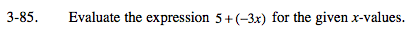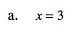When x is placed next to a number, as in this expression, the number is being multiplied by x.

5 + ( −3(x))

Now, substitute 3 for x.
5 + ( −3(3))

Now use the order of operations to simplify this expression.

−4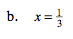Replace x in the expression with one-third.

$5 + \left( -3 \left( \frac{1}{3} \right) \right)$

Simplify using the order of operations. Refer to the Math Notes box of Lesson 3.1.4 if you do not remember how to do so.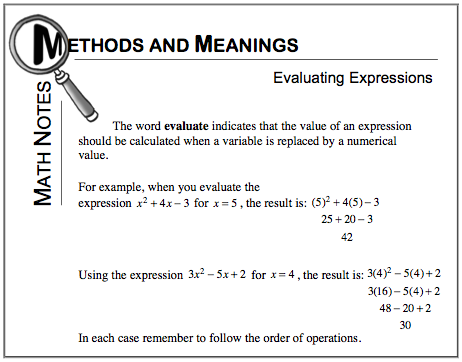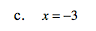See parts (a) and (b) or refer to the Math Notes box of Lesson 3.1.4.